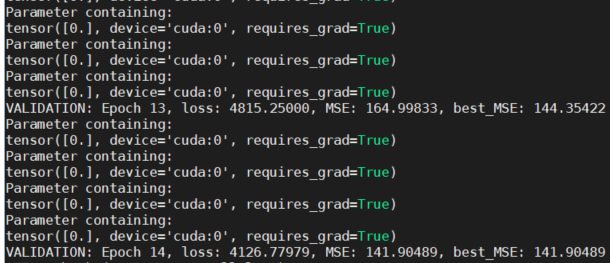# nn.Parameter does not update after training the first fold

Hi,

So I am using 10-fold cross-validation and the model is updated without problems, but after evaluating the first fold the parameter values won’t update. The module I am using is defined below:

``````class CRFlayer(nn.Module, ABC):
def __init__(self, nodes=25):
super(CRFlayer, self).__init__()
self.nodes = nodes
# Sets learnable weights
self.Wh = nn.Parameter(Tensor())
self.Wv = nn.Parameter(Tensor())
self.Wd1 = nn.Parameter(Tensor())
self.Wd2 = nn.Parameter(Tensor())
``````

During the first training I can manually modify the values of the parameters without problem and they will update using something like:

``````with torch.no_grad():
self.Wh.copy_(X1)
self.Wv.copy_(X2)
self.Wd1.copy_(X3)
self.Wd2.copy_(X4)
``````

However, if I use the same code, the weights won’t update during the second iteration of cross-validation (note that as soon as I reach the last epoch of the first iteration, I don’t use the model anymore until the next iteration). I also tried to reset the parameters using this:

``````    elif isinstance(m, CRFlayer):
torch.nn.init.constant_(m.Wh, 0)
torch.nn.init.constant_(m.Wv, 0)
torch.nn.init.constant_(m.Wd1, 0)
torch.nn.init.constant_(m.Wd2, 0)
``````

In both cases, the values are 0 but they don’t change later. I verified the weights using the debugger and the `requires_grad` field is True. Do you have any suggestions? Thanks.

Hey,

No need to call `self.Wh.requires_grad = True`, this is the default when you create a Parameter.

Also you will want to make sure that your params are still properly leaf Tensors after the first update (with .is_leaf()). If they are not, they won’t get their .grad field populated and won’t be updated.
Otherwise, the way you do the update with no_grad and copy_ is the right way to go and won’t cause any issue.

Hi albanD, thanks for the quick response. Yes, the `is_leaf` field is still True after the first iteration. I called `self.Wh.requires_grad = True` just because I wanted to make sure that nothing has changed when I use the model again. The `copy_` works only during the first training but after that, the parameters simply don’t change.

Just as a reference, this shows that the parameters are still 0 after some epochs during the second iteration of the cross-validation.I should mention that I am using two nn.modules. The first is a CNN that is always updated and the other is the CRF layer I showed before. I am using an optimizer that looks like this:

``````params = list(model.parameters())  # CNN parameters
params.extend(list(modelcrf.parameters()))  # Pairwise parameters
``````

I guess something else is problematic then.
Could you give a small code sample (30 lines) that reproduces the issue?

I guess there are too many steps to do something reproducible but the only part where I use the model is this (the problem happes when I execute this for the second time):

``````model = Hyper3DNetLiteReg(img_shape=(1, nbands, windowSize, windowSize))
modelcrf = CRFlayer(nodes=windowSize ** 2)
model.to(device)
modelcrf.to(device)

# Training parameters
criterion = nn.MSELoss()
params = list(model.parameters())  # CNN parameters
params.extend(list(modelcrf.parameters()))  # Pairwise parameters

for epoch in range(epochs):  # Epoch loop
model.network.train()  # Sets training mode
model.crf.train()
running_loss = 0.0
for step in range(T):  # Batch loop
# Generate indexes of the batch
inds = indexes[step * batch_size:(step + 1) * batch_size]
trainb = train[inds]
# Get actual batches
trainxb = torch.from_numpy(trainx[inds]).float().to(device)
trainyb = torch.from_numpy(
np.reshape(train_y[trainb],
(train_y[trainb].shape, 1,
train_y[trainb].shape * train_y[trainb].shape))).float().to(device)
# forward + backward + optimize
outputs = model.network(trainxb)
outputs = torch.reshape(outputs, (outputs.shape, 1, outputs.shape * outputs.shape))
loss = model.crf(outputs, trainyb, self.Lh, self.Lv, self.Ld1, self.Ld2, device, lossC=True)
loss.backward()
model.optimizer2.step()
``````

Is the optimizer here a pytorch provided optimizer?
In general this part of the code is good.

@albanD yes, the optimizer is Adadelta. Fortunately, I found the problem. Each time I finished one iteration, I saved and loaded the best weights using “init.constant_”; replacing it for “copy()” solved the problem.

1 Like